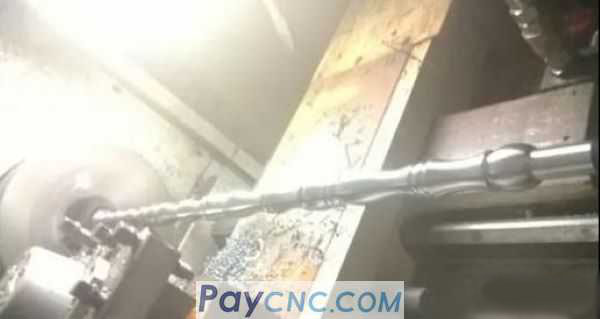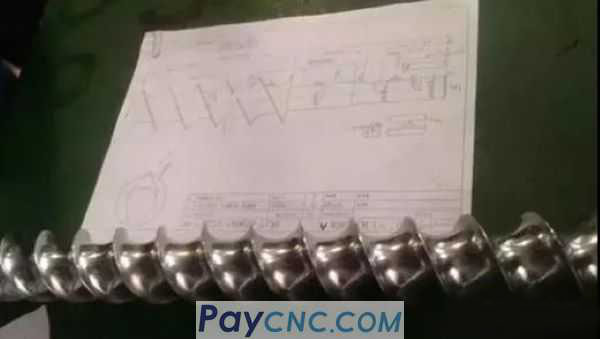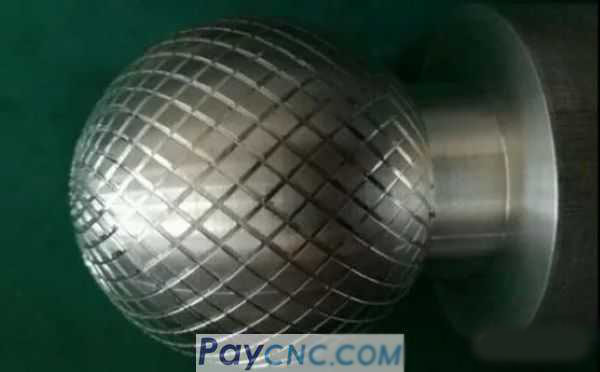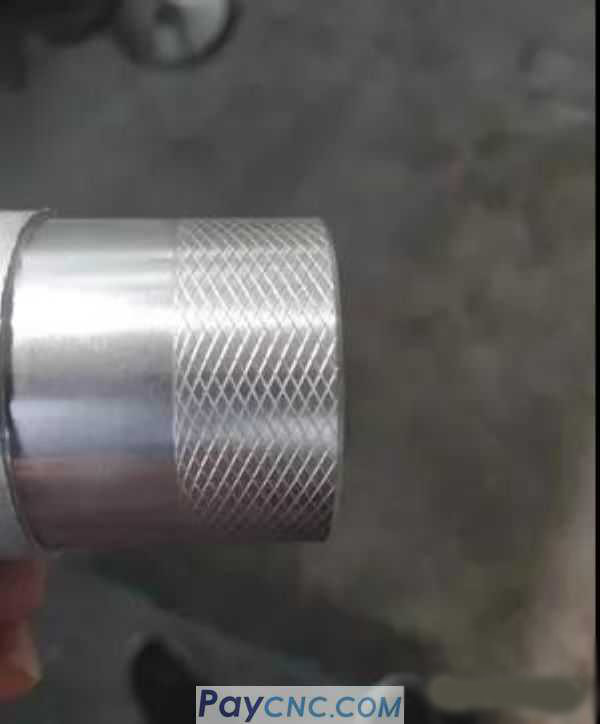How to be a thorough study?   For example: use the macro program to finish the knurling processing on the number car.The experienced master knows: The method of threading, circular indexing, multi-head positive and negative alternating threading, it is the effect of embossing.   Knowing does not mean that they will be. Many masters may be blinded by the implementation of actions.   Let's look at the multi-head thread first, (for example, use the G32 command), the Farak format is as follows:   G32 Z_F_Q_   Among them, Q is the starting angle of the thread. If the starting angle of the single-head thread of the car is zero, it can be omitted.   The main program segments for turning double-end thread are as follows: ... G32Z-50.F30.Q0 (The first head of the car, Q0 can be omitted) ... G32Z-50.F30.Q180000 (the second head of the car)   Remarks: Because it is a double head, a circle of 360 degrees is divided into two, so the starting angle is 0 degrees and 180 degrees. The accuracy unit is 0.001 degree, so Q180000.   If it is 4 threads, the procedure is as follows: ... G32Z-50.F30. ... G32Z-50.F30.Q90000 ... G32Z-50.F30.Q180000 ... G32Z-50.F30.Q270000 ...   What about 8 threads? What about 16 threads?   Obviously only Q is changing, and it changes regularly. Can you think of a certain knowledge point of the macro program at this time?   Is it related to the increment of the variable?   Regarding variable self-increment: for example, set variable #7   Falak’s format is: #7=#7+1, and the +1 at the back means that variable #7 is incremented by 1 every time it is calculated. (Of course, you can also +2 according to your needs, which means that variable #7 will increase by 2 for each operation, or you can also set a variable such as #7=#7+#5, which means that variable #8 will increase the value of #5 every time you operate. ).   Then, when turning a multi-start thread, the change of Q can be changed completely. For example, set the angle variable to #7, and how much does the variable #7 increment each time?   If you want a car with 60 heads, then 360/60=60, that is, Q increments by 60 degrees each time, that is #7= #7+60000   Q. When will it be increased? That is, the multi-threaded 360-degree indexing process is completed.   Can you think of a certain knowledge point of the macro program at this time?   Is it possible to use WHILE []DO statement to control whether to process a circle (360 degrees).   Regarding the WHILE []DO statement, the format is:   WHILE [#7LT#360000] DO 1 ... END 1   When [#7 is less than #360000] the conditional expression is satisfied, the program from DO to END is executed, otherwise, it goes to the program after END.   Then fill in the program content to be processed between DO and END, and fill in the tool withdrawal program after END.   For example, the following part of the program segment: % ……. #7=0 (The initial value of angle variable #7 is zero, and 0 is the starting angle) WHILE[#7LT360000]DO1 (When the value of #7 is less than 360 degrees, execute the program in the loop) G32Z-40.F40.Q#7 G32Z20.F40.Q#7 #7=#7+60000 END1 G0X100 Z100 M30 %   1. The program runs sequentially from top to bottom, and reads #7 data first.   2. Then read the WHILE statement, judge whether the set condition is established, and execute the program from WHILE to END1 if it is established.   3. Because the initial value of #7 is 0, and 0 is less than 360,000, the program from WHILE to END1 is executed. which is:   G32Z-40.F40.Q#7 G32Z20.F40.Q#7   These two sentences happen to be the program of positive and negative threading, and it is a net pattern. #7=#7+60000 (When running to this block, the variable increases automatically, at this time the value of #7 increases by 60000)   4. The machine tool continues to run down to read END1, and then returns to the WHILE statement, again to determine whether the value of #7 is less than 360,000, if it is less than 360,000, continue to execute the program from WHILE to END1.   In this way, the angle index is realized through the increment operation of variable #7, and the WHILE statement controls whether to process a circle (360 degrees).   This is an example of the combined application of variable increment and WHILE statement. After you understand these basic knowledge thoroughly, you can draw inferences about it at any time and use it quickly!   How to draw inferences?   Take another example, for example, the use of macro programs to realize tool life management.   Suppose that my tool can process 200 parts, and the operator should be reminded to change the tool after the accumulated 200 parts are processed.   How to write a program?   The key point here is: when 200 parts are processed cumulatively, the operator should be reminded to change the tool.   Do you think of those knowledge points of macro programs?   Is it possible to use variable auto-increment and WHILE []DO statement to control whether 200 pieces are processed?   For example, the self-increment of variable #501, the format is as follows: #501=#501+1 Through variable #501=#501+1 self-increment, to help me count the number of parts processed   Use the WHILE statement to set conditions: WHILE[#501EQ200]DO1 ... END1 That is, when the value of #501 is equal to 200, the program from WHILE to END is executed.   And I wrote an alarm promotion statement between WHILE and END. When it executes the program between WHILE and END, and reaches the alarm block, the operator will be promoted to change the tool through the alarm screen.   which is: % T0101 S2000 M3 ... (Processing content) ... ... #501=#501+1 WHILE[#501 EQ 200]DO1 #501=0 #3000=1(YOU MUST CHANGE THETOOL) END1 ... M30 %   Remarks: Farak system variable # 3000 is a user-defined alarm variable The format is: #3000=0～200 () When the value of #3000 is 0 to 200, the system will stop running and alarm   Description: 1,0 to 200 is the alarm number, the alarm number displayed on the NC screen is: the value of variable #3000 plus 3000.   2. Alarm information can be written in the brackets, but 26 characters cannot be manipulated in the brackets.   I simply analyze the above program:   1. I put the program paragraph of the part to be processed in front of the WHILE statement, that is, to process the part first. After the part is processed, I will encounter the automatic increment operation of the variable. The value of variable #501 will increase by 1, which is equivalent to the system helping me Count the number of parts processed. The value of #501 will increase by 1 each time the program runs.   2. The setting condition is: when the value of #501 is equal to 200, the block between WHILE and END will be executed.   After the program has been run 200 times, that is, when the value of #501 is 200, the condition is met, and the block between WHILE and END will be executed.   WHILE[#500 EQ 200]DO1 #501=0 #3000=1(YOU MUST CHANGE THETOOL) END1   There are two blocks between WHILE and END. 1, #501=0 2, #3000=1   Adding #501=0 is to clear the value of #501, for the purpose of re-counting after changing the tool. Then when it encounters #3000 block, the program alarms, and the English letters I wrote in parentheses will be displayed in the machine interface to inform the operator to change the tool.   This is another example of the application of analogy.   Well, the example is not important, the analysis method is very important, and the programming idea is very important.